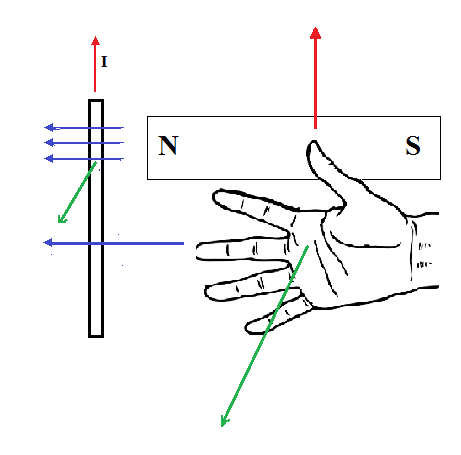Saturday, January 21, 2012

Magnetism: Right Hand Rule #3

Background information
If a current-carrying conductor is placed perpendicular to the magnetic field of a magnet, there will be a force acting on the conductor.

Right Hand Rule #3Red arrows (direction of thumb) represent the direction of current. Blue arrows (fingers) represent the direction of the magnetic field from the bar magnet. Green arrows (direction of palm) represent the direction of force acting on the conductor.

Parallel conductors
When two current-carrying conductors are parallel to each other, they would either attract or repel each other. If the two currents are in the same direction, the two conductors will attract; if the two currents are in opposite directions, the two conductors will repel.

Motor Force
The force that a magnet exerts on a current-carrying conductor can be found using the following equation:
B is the strength of the magnetic field that is perpendicular to the current
I is the current in amperes
L is the length of the conductor (calculated by number of coils multiplied by the length of each coil)